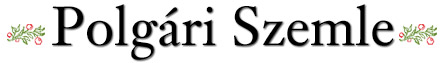The Use of Non-Parametric Criteria for the Evaluation of Similar Patterns in the Behaviour of the Economic System

Polgári Szemle, 13. évf., 4–6. szám, 2017, 380–388., DOI: 10.24307/psz.2017.1228

Inna Strelchenko, PhD, Reader, Department of Economics and Mathematical Modelling, Higher Educational Public Establishment «Vadym Hetman Kyiv National Economic University».

Summary

The statistics made by the International Monetary Fund of a sample of 30 countries analysed to assess the degree of synchronicity of changes under the impact of the crisis on macroeconomic indicators such as increase in: GDP, the exchange rate of the national currency; a country’s international investment position (which characterizes the external liabilities of residents to non-residents); foreign exchange reserves; the value of government bonds. In order to quantify the changes observed, the values of the Kendall concordance coefficient were calculated for equal periods of time – in the crisis and post-crisis periods.

Journal of Economic Literature (JEL) codes: G01, C82, E44, C45
Keywords: financial crises, macroeconomic indicator, classification, rank, coefficient concordance, neural network, Kohonen map

Problem setting

Modern economic policy is required to be able to determine the growth of imbalances in the structure and level of financial markets in a timely manner. In view of the exponentially accelerating pace of economic integration, this task is becoming increasingly complicated. The reason for this is the layering of international relations between goods and money, the increasing pyramid of secondary financial instru-ments, and the shrinking time of cash flow circulations.

When the characteristic features of financial market crises are studied, countries need to be differentiated according to their responses to external financial shocks. The relevance of the topic is highlighted in the Report on Global Financial Stability by IMF.

In order to identify periods when there is similarity (or dissimilarity) in behaviour between the various economic systems, this paper proposes to consider a non-parametric rank correlation test.

Recent research and publications

The relevance of the study of the behaviour of economic systems is unquestionable. The main task of applied research is to describe and then predict the dynamics of development in economic systems. Among the main theoretical concepts it is necessary to distinguish system theory (Bertalanffy, 1968; Nicolis–Prigogine, 1977) and non-linear dynamics (Nicolis–Prigogine, 1977; Derbentsev et al., 2010). As a significant result, research has identified that the particular cases of the complex systems functioning under strictly defined input parameters and constraints on the initial conditions can be formally described.

In this paper, we examine the possibility of studying groups of economic objects in order to identify similarities in their behaviour. To this end, we propose the application of the rank coefficient of concordance (Kendall, 1955; Kendall–Babington Smith, 1939; Legendre, 2005). This was specifically calculated for a test group of thirty countries at different levels of economic development. The calculations were done for two periods: the global financial crisis (01.01.2007-31.12.2009) and the post-crisis period (01.01.2010-31.12.2012). The research objective is to verify the hypothesis that the non-parametric coefficient of rank concordance can be used to classify countries into groups according to the similarity of their response to external financial shocks.

Key research findings

To test the hypothesis of mismatch, the existing IMF classification of countries by level of economic development, for exploring groups of countries by the similarity of responses to external financial shocks, we use the rank coefficient of concordance.

The coefficient of concordance was proposed by M. G. Kendall and B. B. Smith as a measure of the agreement among several quantitative or semi-quantitative variables that are assessing a set of interest objects (Kendall, 1955; Kendall–Babington Smith, 1939; Legendre, 2005):

$W=\frac{12S}{m^2(n^3-n)-mT}\qquad\qquad(1)$

where n is the number of objects and m is the number of variables;

S – is a sum-of-squares statistics over the row sums of ranks Ri:

$S=\sum_{i=1}^n(R_i-\bar{R})^2;\qquad\qquad(2)$

R – is the mean of the Ri values.

The value of the coefficient of concordance ranges from 0 to 1. If W=0, the rank order in rows does not agree (dissimilar). If W=1, the result is interpreted as a full concurrence in the estimates of the studied parameters. Than higher the value of coefficient approaches unity, the higher coherence is observed in the input data series.

In contrast to the procedures of factor or cluster analysis, using the coefficient of concordance in the initial phase of the study has a number of advantages:

• there are no restrictions on the type of distribution of input data;
• there is no need to pre-process the raw data to bring it to a common scale;
• no restrictions are placed on the distribution of grades in the rows of the rank matrix, for example, a normal distribution or linear relationships;
• it has a simple and intuitive interpretation.

In this research the values of the coefficient of concordance evaluate the level of similarity in the dynamics of the selected indicators in crisis and post-crisis periods for the economies of different countries.

The hypothesis about the discrepancy between the existing classification of IMF for the study of groups of countries according to the similarity of the dynamics of response to external financial shocks checks a sample of thirty countries with the represented countries in each group, namely:

1. Advanced Economies: Australia (1), Austria (2), Greece (3), Estonia (4), Israel cted indiator in iis ad pisi piod fr the ecnmie of differ (5), Iceland (6), Canada (7), Germany (8), New Zealand (9), United Kingdom (10) Special administrative region of China Hong Kong (11), South Korea (12), Portugal (13) United States (14), Finland (15) France (16), Czech Republic (17).

2. Emerging and Developing Economies: Brazil (18), Georgia (19), Kazakhstan accoding to the similarity of the dynamics of reponse to external financ (20), Colombia (21), Moldova (22), Paraguay (23) Peru (24), Poland (25), Romania (26th), Turkey (27) Croatia (28), Hungary (29), Ukraine (30).

The statistical data used for calculating the rank coefficient of the Kendall concordance is the official statistical financial report and is publicly available on the official website of the International Monetary Fund.

The consequences of the crisis for the economy of any country can be estimated on the basis of changes in the aggregate the following indicators: GDP, the exchange rate of the national currency, part of the country’s international investment position that characterizes the external liabilities of residents to non-residents, foreign exchange reserves, and the value of government bonds (Matviychuk–Strelchenko, 2015). These macroeconomic indicators are sensitive to financial shocks and show sharp fluctuations in the shortest possible time after the crisis. In order for the results of the evaluation it was possible to compare each indicator should be a relative value and is calculated as the ratio between the current value and value in the previous time:

$x_i=\frac{X_i^t}{{X_i}^{t-1}}\cdot100\%,\qquad\qquad(3)$

where xi – is growth ratio of macroeconomic indicators;

Xit, Xit-1 – is a the absolute value of the macroeconomic indicator at time t and t - 1 respectively;

і = 1,…,5 – is a number of macroeconomic indicator.

A calculating sequence of the ranking coefficient of concordance by the example of a macroeconomic indicator – the quarterly data of the exchange rate in a crisis period (01.01.2007-31.12.2009) (table 1-3) is given below.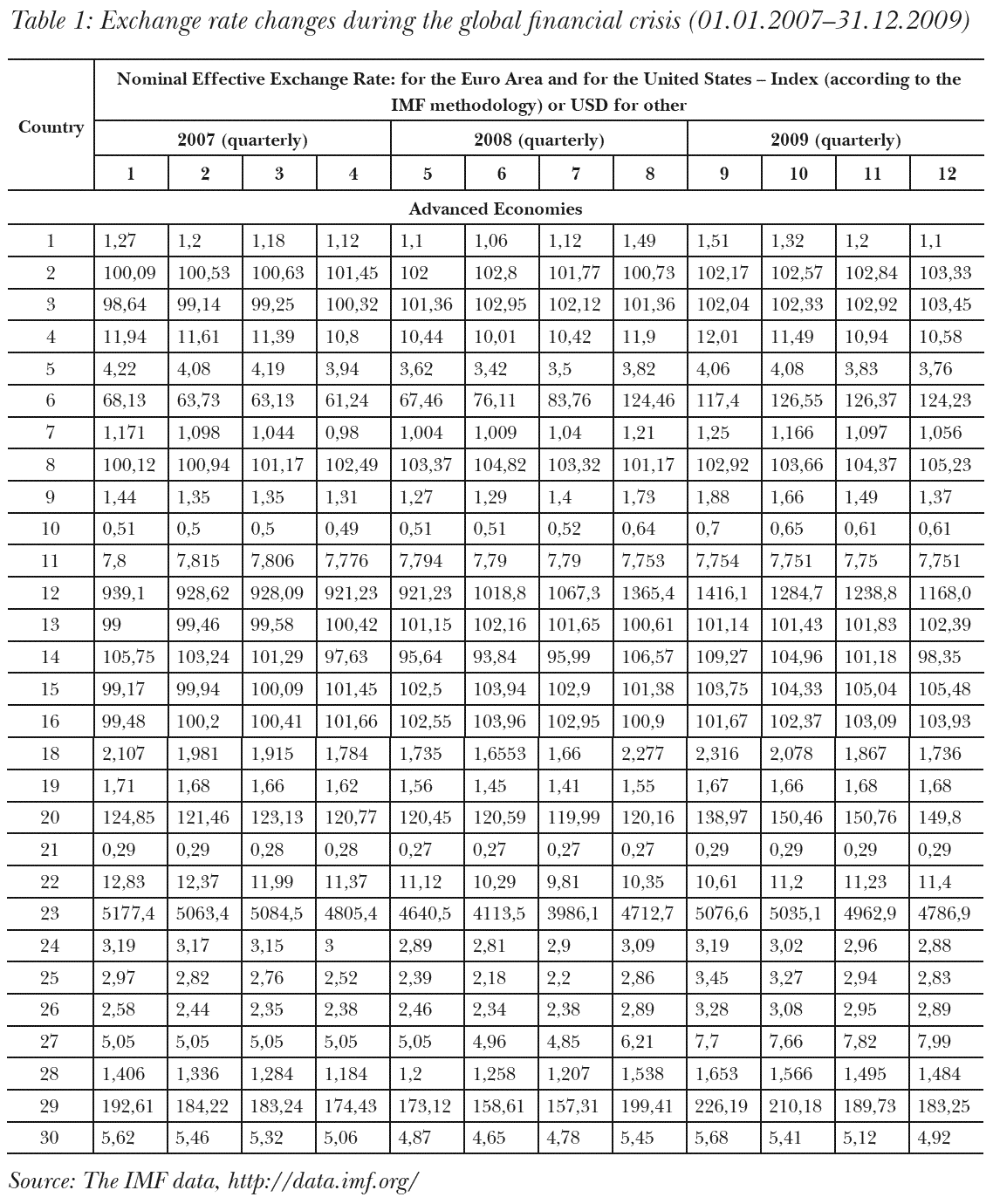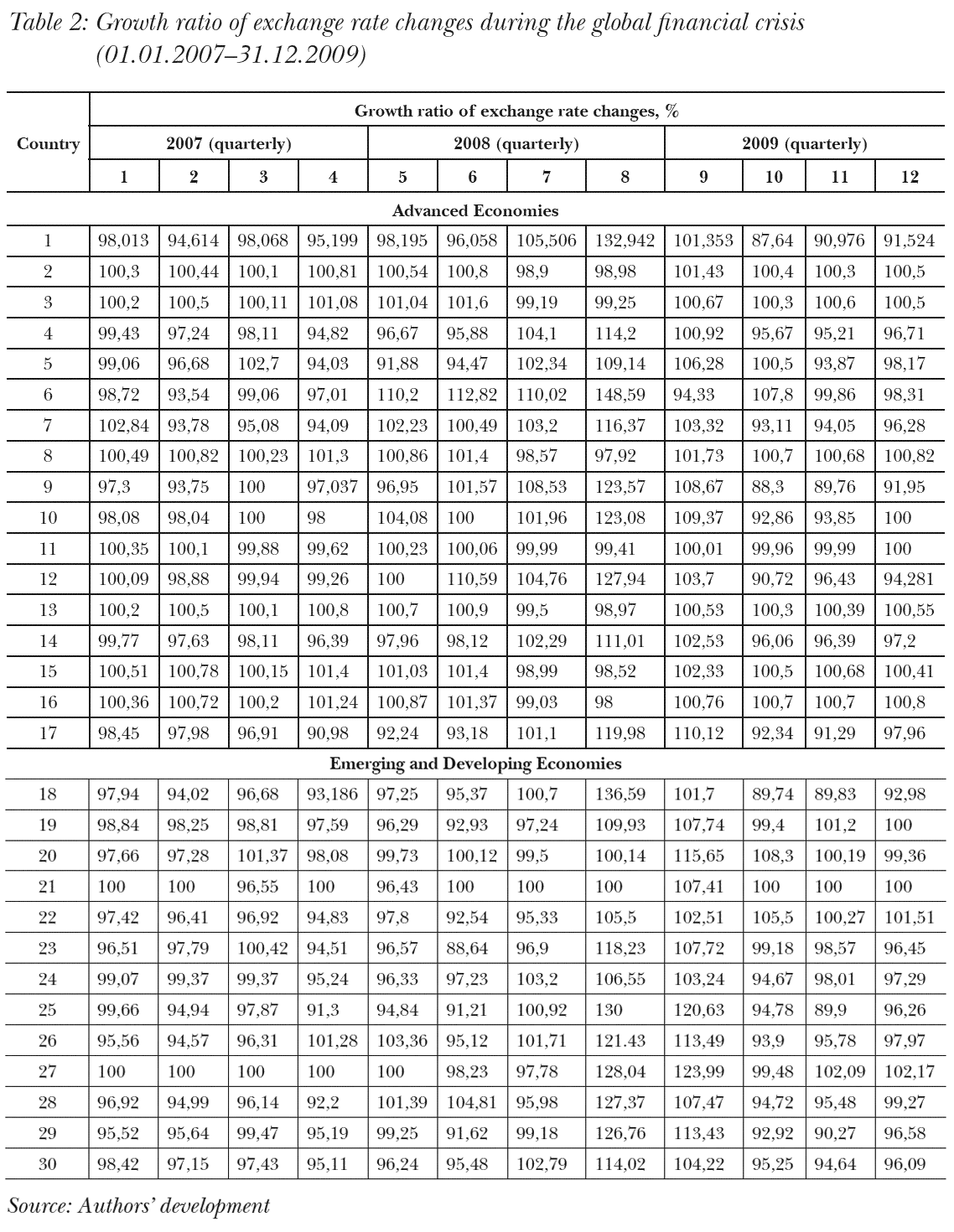In case of tied ranks (see. Table 2) formula to calculate the rank coefficient of concordance is as follows (Kendall, 1955; Kendall–Babington Smith, 1939; Legendre, 2005; Siegel–Castellan, 1988):

$W=\frac{12S}{m^2(n^3-n)-mT}\qquad\qquad(4)$

where T – is correction factor for tied ranks:

$T=\sum_{k=1}^g(t_k^3-t_k),\qquad\qquad(5)$

where tk – is the number of tied ranks in each k of g groups of ties. The sum is computed over all m variables of the input data. (Table 3.)

According to the formula (1-2, 4-5), the coefficient of concordance W is calculated and its significance is determined by Friedman’s chi-square statistics with n-1 degrees of freedom (Legendre, 2005):

$\chi^2=m(n-1)W.\qquad\qquad(6)$

The final results of the assessment of similarity in the dynamics of the selected indicators for the two groups of economies during the global financial crisis of 2007-2009 are shown in Table 4.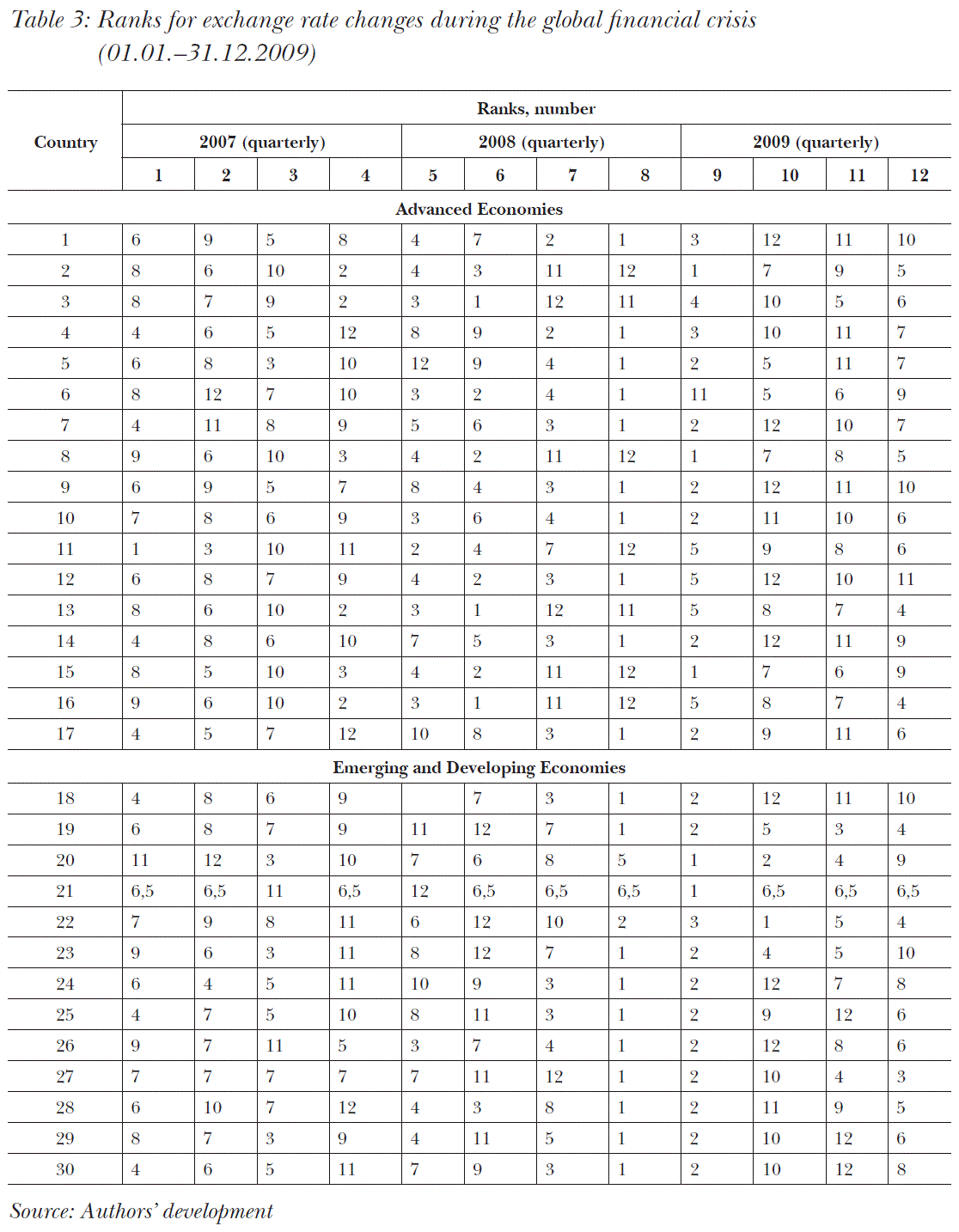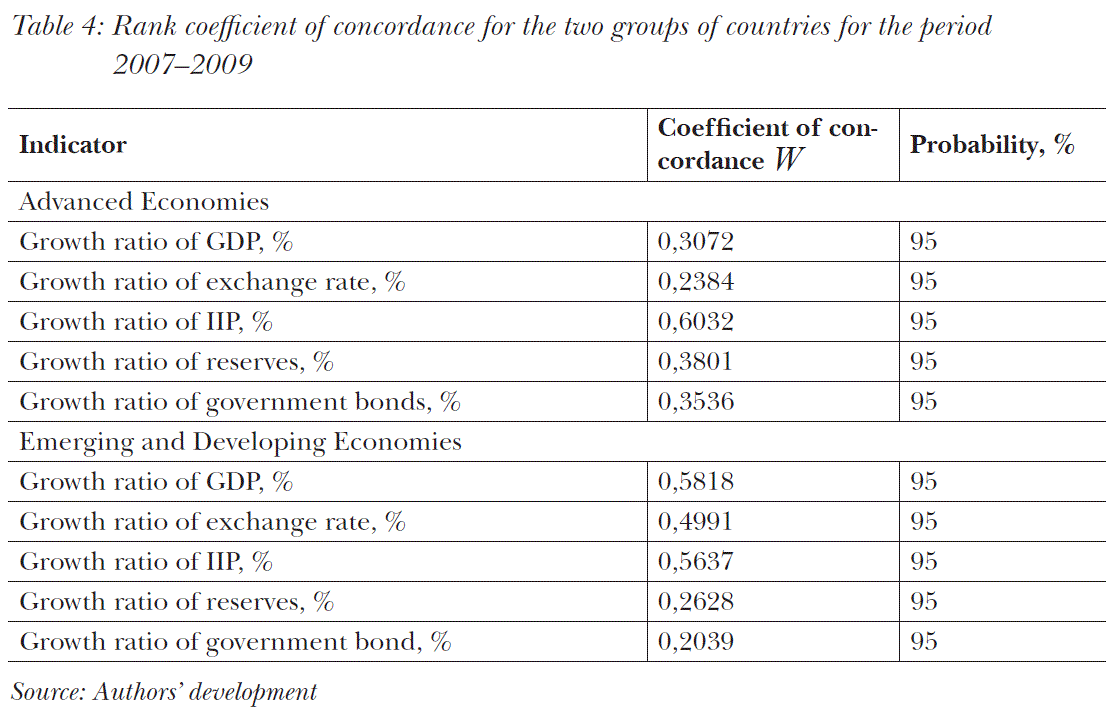The coefficient of concordance is calculated similarly for each indicator for the post-crisis period between 01.01.2010 and 31.12.2012 (Table 5).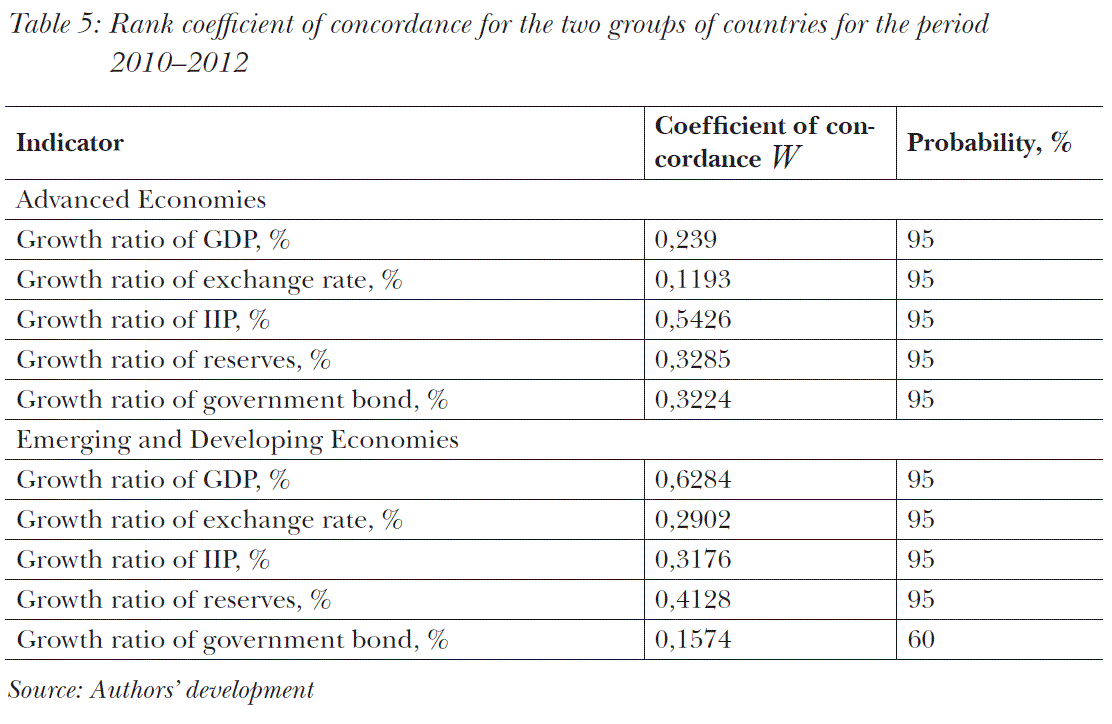On the basis of the obtained results it is highly probable (>95%) that during the global financial crisis (2007-2009) the dynamics of the investigated indicators wass characterized by a low level of similarity (W<0.5 in 80% of cases) for both groups.

Special mention should be made of the results of Table 5, which characterize the post-crisis period (2010-2012). For the group of advanced economies, the value of the concordance coefficient is less than that of the period of the global financial crisis. This indicates a significant difference in the reaction of each economic system to sharp structural changes in the financial sector under the influence of external shocks.

As for groups of countries with emerging markets, it is impossible to make unequivocal conclusions about the more significant differences in the dynamics of indicators in the post-crisis period. In some cases (for example, when calculating the coefficient of concordance for government bonds) the lowest value and a low probability due to the lack of statistics for 35% of the sample countries.

Conclusions

As a result of the studies, the following conclusions were made:

• The reaction of macroeconomic indicators to the course of the financial crisis and the subsequent recovery is significantly different in the economies of different countries;
• The generally accepted classification used by IMF for the level of economic development is unsuitable for solving the practical problem of forecasting the reaction of the economic system to external financial shocks, as evidenced by the results of calculating the rank coefficient of concordance.

Given the specific prerequisites for solving such a problem, for the classification of neural networks it may be reasonable to use instruments like the Kohonen maps or a radial-based architecture.

References

Bertalanffy, Ludwig von (1968): General System Theory. Foundations, Development, Application. George Braziller. Derbentsev V. D. et al. (2010): Synergetchni ta econophizichni metody doslidzhennya dynamichnykh ta strukturnykh kharakteristyk ekonomichnych system. / Brama.
IMF: Statistical information according to International Monetary Fund. http://data.imf.org/.
Kendall, Maurice G. (1955): Rank Correlation Methods. Hafner Publishing Co.
Kendall, Maurice G. – Babington Smith, B. (1939): The Problem of m Rankings. The Annals of Mathematical Statistics, No. 10, 275-287, http://dx.doi.org/10.1214/aoms/1177732186.
Legendre, Pierre (2005): Species Associations: The Kendall CoefficientofConcordance.JournalofAgricultural, Biological & Environmental Statistics, Vol. 10, No. 2, 226-245, https://doi.org/10.1198/108571105x46642.
Matviychuk, A. V. – Strelchenko, I. I. (2015): Vykorystannya systemy vyperedzhauchikh indykatoriv dlya prognozuvannya negatyvnykh zrushen’ na finansovomu rynku Ukrainy. Finansy Ukrainy, No. 8, 74-87, http://nbuv.gov.ua/UJRN/Fu_2015_8_7.
Nicolis, Gregoire – Prigogine, Ilya (1977): Self-Organization in Nonequilibrium Systems. From Dissipative Structures to Order Through Fluctuations. Wiley.
Siegel, Sidney – Castellan, N. John (1988): Nonparametric Statistics for the Behavioral Sciences. McGraw-Hill, Singapore.
© 2005 – 2019 Polgári Szemle Alapítvány